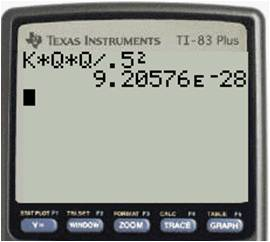## Storing constants on your graphing calculator

There are several fundamental constants in physics that you will use over and over. If you get tired of looking them up every time you need one for a calculation, consider storing them in your calculator. The graphing calculator makes it easy to store any value as one of the alphabet letters. It then remains in the calculator until you remove it.

To store a number, type it into the calculator, press the Store key (STO→), then select a letter with the ALPHA key. Then when you need the value, just type the letter on your calculator. Here are the constants I store in my calculator:

 Constant letter value Coulomb law constant k 8.99E9 Elementary charge q 1.60E-19 Planck’s constant h 6.63E-34 speed of light c 2.998E8 Universal gravitation constant G 6.67E-11

Other constants you might find useful to store are proton mass, electron mass, vacuum permittivity and permeability, and Boltzmann’s constant.

For example, to calculate the electrical repulsion force between two electrons that are fixed 0.5 m apart, use the equation$\displaystyle F = \frac{kq_{1}q_{2}}{r^2}.$

Because the values of$k$ and$q$ are already stored in my calculator, here’s the way this would appear when I crunch it:Blue Taste Theme created by Jabox